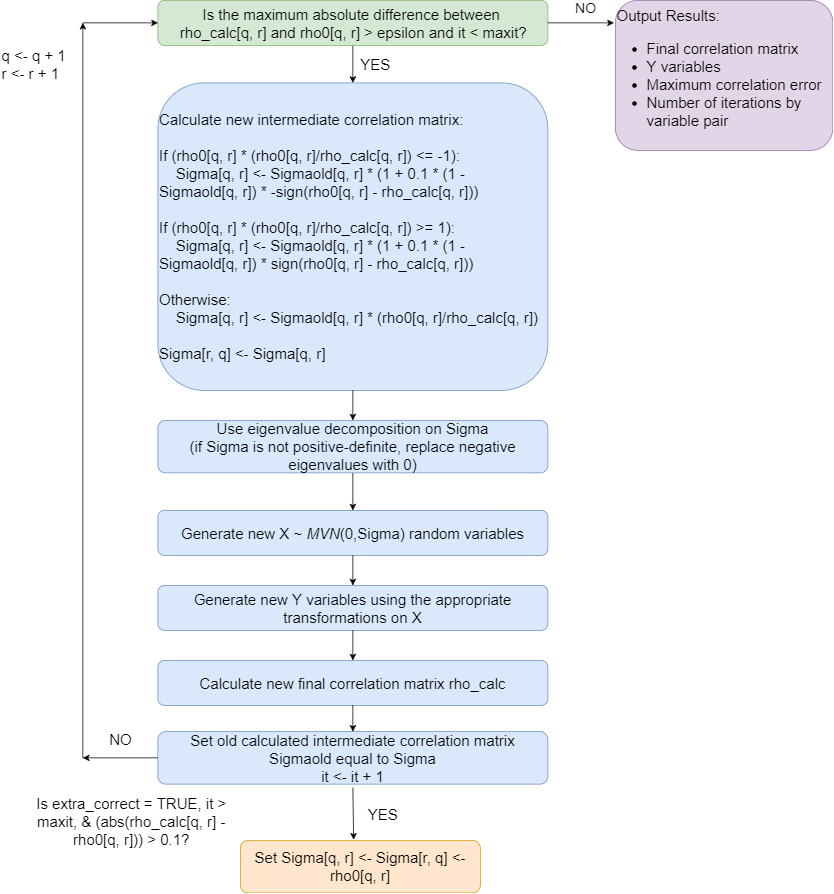Overview of Error Loop

2018-06-28

The error loop may be used to correct the final correlation of simulated variables to be within a user-specified precision value (epsilon) of the target correlation. It updates the pairwise intermediate MVN correlation iteratively in a loop until either the maximum error is less than epsilon or the number of iterations exceeds the maximum number set by the user (maxit). It uses SimMultiCorrData::error_vars to simulate all the variables in each iteration. The function modifies Barbiero and Ferrari (2015) ordcont function in the GenOrd package in the following ways:

1. It works for continuous, ordinal ($$\Large r \ge 2$$ categories), and count variables.
2. The initial positive-definite correlation check has been removed because the intermediate correlation Sigma from rcorrvar or rcorrvar2 has already been used to generate variables (and therefore is positive-definite).
3. Eigenvalue decomposition is done on Sigma to impose the correct intermediate correlations on the normal variables. If Sigma is not positive-definite, the negative eigenvalues are replaced with 0.
4. The final positive-definite check has been removed.
5. The intermediate correlation update function was changed to accommodate more situations.
6. An optional final “fail-safe” check was added at the end of the iteration loop where if the absolute error between the final and target pairwise correlation is still > 0.1, the intermediate correlation is set equal to the target correlation (if extra_correct = “TRUE”)
7. Allowing specifications for the sample size and the seed for reproducibility.

The error loop does increase simulation time, but it can improve accuracy in most situations. It may be unsuccessful in more difficult to obtain correlation structures. Some cases utilizing negative correlations will have results similar to those without the error loop. Trying different values of epsilon (i.e., 0.01 instead of 0.001) can help in these cases. For a given row (q = 1, …, nrow(Sigma)), the error loop progresses through the intermediate correlation matrix Sigma by increasing column index (r = 2, …, ncol(Sigma), r not equal to q). Each time a new pairwise correlation Sigma[q, r] is calculated, the new Sigma matrix is imposed on the intermediate normal variables X, the appropriate transformations are applied to get Y, and the final correlation matrix rho_calc is found. Even though the intermediate correlations from previous q, r combinations are not changed, the final correlations are affected. The fewer iterations for a given q, r combination, the less rho_calc[q, r] changes. Since larger values of epsilon require fewer iterations, using epsilon = 0.01 may give better results than epsilon = 0.001.

Below is a schematic of the algorithm. In the figure, rho_calc is the calculated final correlation matrix updated in each iteration, rho0 is the target final correlation matrix, Sigmaold is the intermediate correlation matrix from the previous iteration, it is the iteration number, q is the row number, and r is the column number. In addition, extra_correct refers to the setting in the simulation functions (see rcorrvar and rcorrvar2).Overview of Error Loop.